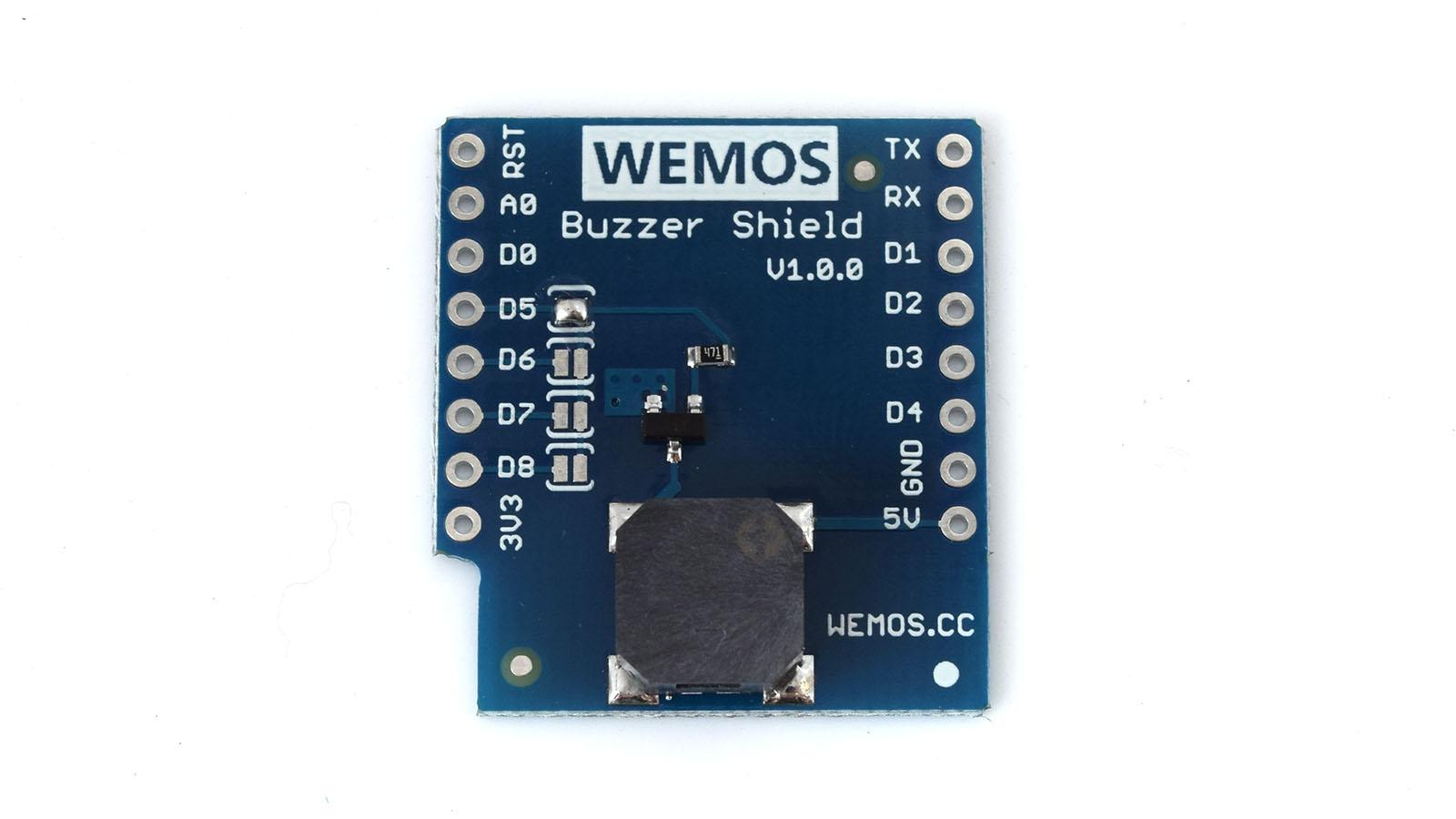# Wemos buzzer shield example

In this example we look at another Wemos mini shield, this time its the buzzer_shield which as its name suggests is a basic buzzer. Its an MLT_8540.

The product page is https://wiki.wemos.cc/products:d1_mini_shields:buzzer_shieldCode

This example plays a few notes from a popular song

 Source code```int buzzerPin=D5; //Buzzer control port, default D5
const int songLength = 18;
char notes[] = "cdfda ag cdfdg gf ";
int beats[] = {1,1,1,1,1,1,4,4,2,1,1,1,1,1,1,4,4,2};
int tempo = 150;

void setup()
{
pinMode(buzzerPin, OUTPUT);
}

void loop()
{
int i, duration;

for (i = 0; i < songLength; i++) // step through the song arrays
{
duration = beats[i] * tempo;  // length of note/rest in ms

if (notes[i] == ' ')          // is this a rest?
{
delay(duration);            // then pause for a moment
}
else                          // otherwise, play the note
{
tone(buzzerPin, frequency(notes[i]), duration);
delay(duration); // wait for tone to finish
}
delay(tempo/10);              // brief pause between notes
}

while(true){}

}

int frequency(char note)
{

int i;
const int numNotes = 8;  // number of notes we're storing
char names[] = { 'c', 'd', 'e', 'f', 'g', 'a', 'b', 'C' };
int frequencies[] = {262, 294, 330, 349, 392, 440, 494, 523};

for (i = 0; i < numNotes; i++)  // Step through the notes
{
if (names[i] == note)         // Is this the one?
{
return(frequencies[i]);     // Yes! Return the frequency
}
}
return(0);
}```

1.Coder58
•shedboy71